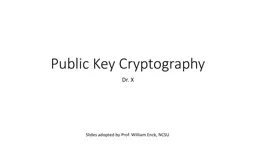# Public Key Cryptography Dr. XEmbed code:

## Public Key Cryptography Dr. X

Download Presentation - The PPT/PDF document "Public Key Cryptography Dr. X" is the property of its rightful owner. Permission is granted to download and print the materials on this web site for personal, non-commercial use only, and to display it on your personal computer provided you do not modify the materials and that you retain all copyright notices contained in the materials. By downloading content from our website, you accept the terms of this agreement.

### Presentations text content in Public Key Cryptography Dr. X

Slide1

Public Key Cryptography

Dr. X

Enck

, NCSU

Slide2

Private-key crypto is like a door lock

Slide3

Encryption and Message Authenticity

Slide4

Public Key Crypto (10,000

ft view)

Separate keys for encryption and decryption

Public key: anyone can know this

Private key: kept confidential

Anyone can encrypt a message to you using your public key

The private key (kept confidential) is required to decrypt the communication

Alice and Bob no longer have to have

a priori

shared a secret key

Slide5

Public Key Cryptography

Each key pair consists of a public and private component:

k+ (public key), k- (private key)

D

k

-

(

E

k

+

(m)) = m

Public keys are distributed (typically) through public key certificates

Anyone can communicate secretly with you

Slide6

Modular Arithmetic

Integers Z

n

= {0, 1, 2, ..., n-1}

x mod n = remainder of x divided by n

5 mod 13 = 5

13 mod 5 = 3

y is

modular inverse

of x

iff

xy

mod n = 1

4 is inverse of 3 in Z

11

If n is prime, then Z

n

has modular inverses for all integers except 0

Slide7

RSA (

The dominant public key algorithm

The algorithm itself is conceptually simple

Why it is secure is very deep (number theory)

Uses properties of exponentiation modulo a product of large primes

Slide8

Euler’s Totient Function

coprime

: having no common positive factors other than 1 (also called

relatively prime

)

16 and 25 are coprime

6 and 27 are not coprime

Euler’s Totient Function

: Φ(n) = number of integers less than or equal to n that are coprime with n

where product ranges over distinct primes dividing n

If m and n are coprime, then Φ(mn) = Φ(m)Φ(n)

If m is prime, then Φ(m) = m - 1

Slide9

Euler’s Totient Function

Slide10

RSA Key Generation

Choose distinct primes p and q

Compute n =

pq

Compute

Φ

(n) =

Φ

(

pq

) = (p-1)(q-1)

WHY?

Randomly choose 1<e< Φ(

pq

) such that e and Φ(pq) are coprime. e is the public key exponent Compute de=1 mod(Φ(pq

)).

d is the private key exponent Example: let p=3, q=11, n=33 … find e, d

Slide11

Public Key Encryption & Decryption

Public key k

+

is {

e,n

} and private key k

-

is {

d,n

}

Encryption and Decryption

Ek

+

(M) :

ciphertext

= plaintexte mod n Dk-(ciphertext) : plaintext = ciphertext

d

mod n ExamplePublic key (7,33), Private Key (3,33) M = 4 …

Slide12

Why does it work?

Difficult to find

Φ

(n) or d using only e and n.

Finding d is equivalent in difficulty to factoring n as p*q

No efficient integer factorization algorithm is known

Example: Took 18 months to factor a 200 digit number into its 2 prime factors

It is feasible to encrypt and decrypt because:

It is possible to find large primes.

It is possible to find co-primes and their inverses.

Modular exponentiation is feasible.

Slide13

Is RSA secure?

{e,n

} is public information

If you could factor

n

into

p*q,

then

could compute

φ

(

n

)

=

(

p-1)(q-1)could compute d = e-1 mod φ(

n

)would know the private key <d,n>! But: factoring large integers is hard!classical problem worked on for centuries; no known reliable, fast method

Slide14

RSA Security

At present, key sizes of 1024 bits are considered to be secure, but 2048 bits is better

Tips for making

n

difficult to factor

1. p

and

q

lengths should be similar (ex.: ~500 bits each if key is 1024 bits)

2. both (

p

-1) and (

q

-1) should contain a “large” prime factor

3.

gcd(p-1, q-1) should be “small” 4. d should be larger than

n

1/4

Slide15

Attacks Against RSA

Brute force: try all possible private keys

can be defeated by using a large enough key space (e.g., 1024 bit keys or larger)

Mathematical attacks

1. factor

n

(possible for special cases of n)

2. determine

d

directly from

e,

without computing

φ

(

n

) – at least as difficult as factoring n http://crypto.stackexchange.com/questions/3043/how-much-computing-resource-is-required-to-brute-force-rsa

Slide16

Attacks

Probable-message attack (using {

e

,

n

})

encrypt all possible plaintext messages with {e, n}

Intercept a message (ciphertext)

try to find a match between the ciphertext and one of the encrypted messages (i.e., collision!)

only works for small plaintext message sizes

This can intercept a secret key that was sent with public key crypto

Solution: pad plaintext message with random text before encryption

Slide17

Timing Attacks Against RSA

Recovers the private key from the running time of the decryption algorithm

Computing m =

c

d

mod

n

using repeated squaring algorithm:

Slide18

Timing Attacks

The attack proceeds bit by bit

Attacker assumed to know

c

,

m

• Attacker is able to determine bit

i

of

d

because for some

c

and

m

, the highlighted step is extremely slow if

di =1 http://www.cs.sjsu.edu/faculty/stamp/students/article.html

Slide19

Countermeasures to Timing Attacks

1. Delay the result if the computation is too fast